# More On Frequency Modulation

Last month, we examined the frankly scary maths allowing you to predict the audible effects of Frequency Modulation. This time, although the maths gets even tougher, Gordon Reid relates the theory to the practical implementation of FM synthesis on Yamaha's digital synths, as well as modular and non-modular analogues.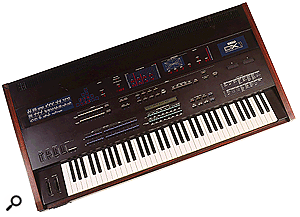Yamaha's flagship FM synthesizer, the DX1.After reading last month's part of this series, you will (I hope) have progressed a fair way towards understanding Frequency Modulation. Nevertheless, if you were now presented with a digital FM instrument or a modular analogue synth patched to allow frequency modulation, the chances are that you would still make FM noises rather than musically-pleasing timbres.

This is because we have not yet discussed the nature of the relationship between the side bands (which are produced as a side-effect of the actual process of Frequency Modulation itself) and the Carrier (the signal being modulated). Nor have I explained how these relationships can be made to fit the simple harmonic theories explained right at the start of this series, which would allow you create tones that human ears would perceive as 'notes'. Furthermore, apart from a couple of basic diagrams, I have made no attempt to show you how to control the amplitudes of the side bands. So hang on to your hats, because this is where the fun (umm... headache?) really begins.

## Introducing Carrier:Modulator (C:M) Ratios

We already know that each side band in a Frequency Modulated signal lies at a frequency equal to the Carrier frequency plus or minus an integer multiple of the Modulator frequency. I expressed this last month as follows: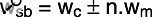Equation 1: The maths for working out the frequencies of the side bands produced by frequency modulation.

In this equation, wsb = the series of side band frequencies, wc = the frequency of the Carrier, wm = the frequency of the modulator, and n = any integer (0, 1, 2, 3, 4...).

Now I'm going to risk complicating matters by eliminating references to frequencies in Hertz. We can express the relative frequencies of Carrier and Modulator using a ratio that I will refer to as the 'C:M Ratio'. For example, if the Carrier frequency wc is 100Hz and the Modulator frequency wm is 200Hz, I will refer to these as frequencies with a 1:2 ratio. I will then use C and M to refer to the Carrier and Modulator frequencies as they are expressed in the C:M ratio — what matters is the relative frequencies of Carrier and Modulator, not their absolute values in Hz.

Having made this definition, we can now say that, for any given Carrier frequency, the frequencies of the 'upper' side bands lie at C+M, C+2M, C+3M, C+4M... while those of the 'lower' side bands lie at C-M, C-2M, C-3M, C-4M... and so on. This is an important result, because it immediately associates itself with the idea of harmonic series.

Imagine an example where the C:M ratio is 1:1. In this case, the Carrier lies at '1', and the 'upper' side bands lie at '2', '3', '4'... while the 'lower' side bands lie at '0', '-1', '-2', '-3', '-4'... and so on. Now, if wc is 100Hz, then wm must also be 100Hz (it is a 1:1 ratio), and the upper side bands must therefore lie at 200Hz, 300Hz, 400Hz... ad infinitum. This, of course, is a perfect harmonic series for a 100Hz wave. In other words, the upper side bands of a 1:1 C:M ratio produce a harmonic series no matter what the Carrier frequency may be.

But what about the lower side bands? If we extend our argument, these lie at 0Hz, -100Hz, -200Hz, -300Hz... also ad infinitum. Hang on... what are negative frequencies?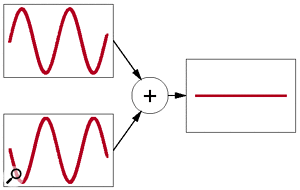Figure 1: The cancellation of out-of-phase sine waves.It turns out that there is a simple answer to this. Negative frequencies are the same as positive frequencies, but with their phases inverted. Yet, as we showed in Part 4 of this series, out-of-phase signals will cancel out, leaving silence (see Figure 1). So surely the 1:1 ratio will result in silence as the 100Hz component cancels the -100Hz component, 200Hz cancels -200Hz ... and so on?

The answer, fortunately, is 'no', and the reason for this is simple: for total cancellation to occur, the amplitudes of the cancelling components must be equal, and in this case they are not. So how do we know what the amplitudes of the side bands will be?

## Enter The Bessel Functions

If you've been reading this series from the start, you'll have noticed that the term 'Bessel Function' has cropped up several times. This is because Bessel functions are a family of equations that describe some fundamental characteristics of the universe in which we find ourselves. Just as the value of Pi (3.141592654... and so on and so on) is much more than simply something to do with the circumferences of circles, Bessel functions crop up in almost every aspect of maths, physics and engineering. What's more, they are the key to FM because, if you know the value of the Modulation Index (as discussed last month), you can calculate the amplitude of any spectral component using a Bessel Function.

Now, if you want to skip the next few paragraphs, I wouldn't blame you in the slightest, particularly if you're not a fan of maths. What's more, leaving this next bit out won't interfere with your understanding of what comes afterwards. But the more adventurous among you may like to read on...

Let's define a couple of things that will make life simpler. Let's call the Carrier 'C' the 'zeroth-order' components of our modulated signal, 'C+M' and 'C-M' the first-order components, 'C+2M' and 'C-2M' the second-order components... and so on.

Having done this, I can tell you (but not prove without recourse to a serious maths text book) that the amplitude of each pair of side bands of order 'n' (remember, n is any whole number from 0 to infinity) is defined by a Bessel function of order 'n'. I have shown this in Equation 2.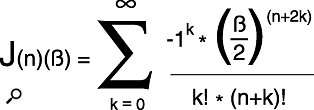Equation 2: The mathematical nightmare that is a Bessel Function!

In this equation, J(n)(ß) is the nth-order Bessel Function for any Modulation Index ß, k is just an integer 0, 1, 2, 3, 4... up to infinity, ! is the algebraic term for 'factorial' (if you don't understand this, don't worry about it), and (sum) means 'the sum of all the terms from k=0 to k=(infinity).

Go on, admit it... this is your worst nightmare. What does this have to do with synthesis and making music? Well, quite a lot, actually. Every time you program a DX synthesizer you're using this. It's just that Yamaha have (kindly) hidden it from view. More importantly, every time you use the 'Cross Modulation' function on analogue synths that are lucky enough to have one, the nasty noises you create are determined by this equation — cross modulation being, of course, just another name for frequency modulation.

Anyway, if you'll bear with me for a little longer, I'll show you that Equation 2 isn't actually quite as bad as it looks. Imagine the modulation index ß is 0.1, and you want to calculate the amplitude of the zeroth component (ie. the Carrier). We can calculate the first term (when k=0) as shown in Equation 3, and the second term (k=1) as shown in Equation 4.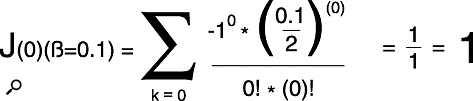Equation 3: The first term in calculating the amplitude of the Carrier.

Equation 4: The second term in calculating the amplitude of the Carrier.

You now do this for all the remaining terms (k=2, k=3, k=4... and so on) and then add them all up. If you could be bothered to do this, you would find that the third term is even smaller than the second (and positive), the fourth term is even smaller than that and negative, and so on. This is called a converging series, and if you add up all of its terms, it demonstrates that the amplitude of the Carrier remains very close to 1, its initial amplitude. In principle, this calculation should take an infinite amount of time because k represents every whole number from 0 to infinity — but fortunately, anything beyond the third term is so tiny that it is irrelevant.

OK, so that's the amplitude of the Carrier. Now, what of the side bands? To work out the amplitudes of the first order side bands C+M and C-M, you just substitute n=1 in Equation 2, reset k to zero, and start the whole process again. When you have done this, you substitute n=2 in Equation 2, reset k to zero again, and calculate the amplitudes of the second-order side bands C+2M and C-2M. Then you substitute n=3, n=4... and so on, and on, and on... As you can see, you have an infinite series of infinite series to calculate, so I'll keep a table reserved for you at the Restaurant At The End Of The Universe. (Unfortunately, you'll be late.) Oh yes, and when you've finished that, Wiggins Minor, you can start on ß=0.5 for me. Argghh!!!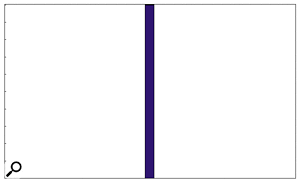Figure 2: The amplitudes of the spectral components when ß=0.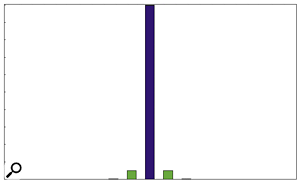Figure 3: The amplitudes of the spectral components when ß=0.1.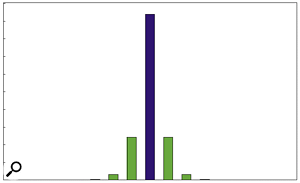Figure 4: The amplitudes of the spectral components when ß=0.5.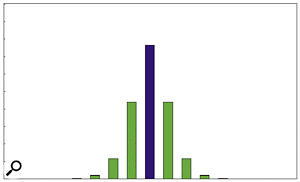Figure 5: The amplitudes of the spectral components when ß=1.Fortunately, there are powerful numeric methods that will calculate all these amplitudes for you. Even the common (but very powerful) Microsoft Excel will give you the values of any Bessel function of order n and Modulation Index ß. I have, therefore, created a simple spreadsheet to generate the amplitude charts, shown as Figures 2 and Figures 3 to 7. These include the Carrier (the zeroth order component, in blue) and the first eight components (in green) on either side.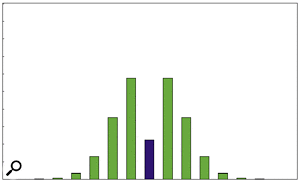Figure 6: The amplitudes of the spectral components when ß=2.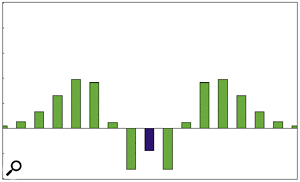Figure 7: The amplitudes of the spectral components when ß=5.As you can see from Figure 7, the amplitudes of the Carrier and first-order side bands are negative when ß=5. This does not mean that these frequencies are, by some mathematical sorcery, transported into a negative universe, it means that they are present in the output but with inverse phase.

## Creating Recognisable Harmonic Series

Let's now return to our example of a 1:1 series with ß=1. Looking at Figure 5 you can see that the Carrier retains much of its original amplitude, and that — looking to its right — there is a harmonic series at 2C, 3C, 4C and 5C. Now, looking to the left, we see that there is a significant component (C-M) at 0Hz (known as a DC or direct current component, because it has no oscillation frequency, as discussed in Part 11 of this series), and low-amplitude components at -C, -2C and -3C. These, as already discussed, reflect with inverse phase to C, 2C and 3C, and are therefore subtracted from the in-phase components at the same frequencies — see Figure 8 (below). If we ignore the DC component, the resulting spectrum contains all the overtones of the Carrier frequency (1:1 is the only ratio that does this) and it looks like a 1/n harmonic series with just the first few harmonics present. If you remember the first part of this series, you'll recall that this describes a filtered sawtooth wave perfectly! And sure enough, that's exactly how it sounds.

Now let's consider the case of a 1:2 frequency modulation with ß=1. In this case the upper side bands exist at C, 3C, 5C, 7C... and so on, while the subtractive negative components reflect back at C, 3C, 5C, 7C... and so on. So, in this case, the result is a truncated harmonic series with just the odd harmonics present. Does this seem familiar? It should, because it's what you get when you filter a square wave! And, once again, that's how it sounds.

Moving on, what about C:M ratios of 1:3, 1:4, and other integers? Given what we've already discussed, you don't need diagrams to work these out. The ratio 1:3 gives upper side bands at 4C, 7C, 10C... and reflected lower side bands at 2C, 5C, 8C... which (ignoring phase inversion) is reminiscent of the spectrum of a 33 percent pulse wave. Similarly, 1:4 produces upper side bands at 5C, 9C, 13C... and reflected lower side bands at 3C, 7C, 11C... which is again similar to that of a square wave.

By the way, this explains why there were 24 spectral components in last month's example of an output with 3600Hz bandwidth. There were 10 upper side bands in the signal, the Carrier, one unreflected lower side band, and 12 reflected lower side bands.

At this point, you might be thinking that there's nothing too complicated about this FM malarkey (apart from maybe the maths), but like most things in life, it's not as straightforward as it might appear. The integer C:M ratios are relatively straightforward because they produce harmonic waveforms with the fundamental (which, by the way, may not be the loudest component) at the Carrier frequency. But these are special cases, and there is an infinite range of non-integer ratios from which to choose. I'll leave it to you to work out the spectrum of, for example, a 1:1.2138754 C:M ratio but, clearly, the resulting sound will be completely enharmonic. Not only that, but the Carrier will no longer be the lowest frequency in the spectrum.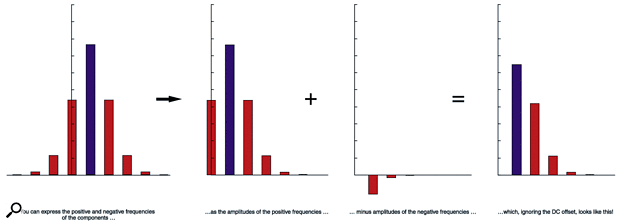Figure 8: The spectrum of a 1:1 frequency modulation with ß=1.

## FM Synthesis On Analogue Synthesizers

Some analogue polysynths such as the Oberheim Matrix 6 are capable of simple, 2-oscillator FM. This is because their digitally controlled oscillators (DCOs) are stable enough to maintain the precise frequency ratios required for the technique (see last month's instalment of Synth Secrets for more on this). However, FM is only truly versatile when you have access to a large number of oscillators, VCAs and contour generators. Furthermore, however many modules you need for a sound, you will need twice as many for duophony, three times as many for 3-note polyphony... This soon becomes a very large number of modules, and is undoubtedly the reason why FM never caught on in the analogue realm. Just consider the size of the modular synth that you would need to emulate a DX7: 96 oscillators, 96 VCAs and 96 multi-stage EGs for the oscillator levels, plus pitch envelope generators and their associated VCAs, innumerable mixers, and heaven-knows-what-else. The total system would incorporate hundreds of modules and would weigh many tons.

Despite this, you can still produce interesting monophonic FM sounds using just a handful of analogue modules. What's more, these need not be part of a modular synth; they could just as easily be the sections within a pre-patched monosynth.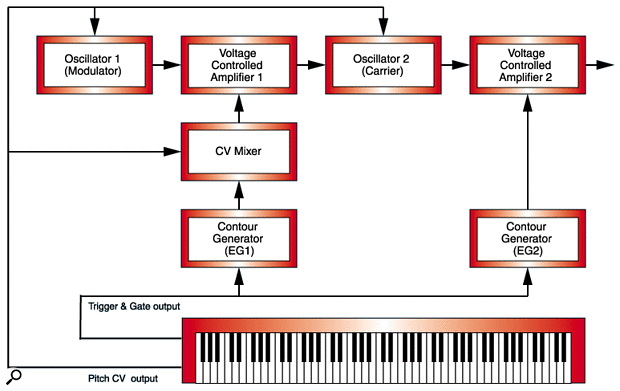Figure 9: Simple FM on a modular analogue synth.

Take a look at Figure 9. This shows a basic 2-oscillator FM configuration that uses just seven modules. Now we'll assign some numbers to the modules. Let's tune both OSC1 and OSC2 to the same frequency for a 1:1 ratio. Now set the amount of EG1 to zero (so that it is 'off'), and define EG2 to be a 0/0/10/0 (no attack, no decay, full sustain, instantaneous release) organ-type envelope. If you play a note, you will hear something that sounds similar to a sawtooth wave, as described above. Furthermore, you can fine-tune the pitch and the timbre by adjusting the gain of VCA1.

Having done this, you can now play with the gain of VCA1 and apply contours from EG1 to create radical timbral changes that often sound like nothing so much as sci-fi sound effects. If you now repeat this exercise with OSC2 set to twice the frequency of OSC1, your basic waveform will now sound more 'hollow', and much like a square wave.

Of course, you can easily let your imagination run riot, and use all manner of unrelated frequencies as starting points, or use scaled CVs to make the oscillators track at different rates. These will give you wildly varying sounds, all of which will be far more complex that those created simply by mixing the outputs of any two analogue oscillators. Indeed, with appropriate enveloping of the Carrier output, these sounds can be excellent for creating drums and other powerful percussion effects.

## Operators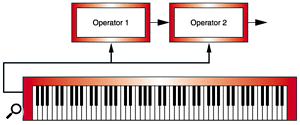Figure 10: Another way of drawing Figure 9... this time as a '2-operator' FM synthesizer.Unfortunately, block diagrams such as Figure 9 become very complex very quickly, so we need a shorthand way to represent them. We do this by introducing the concept of an operator — a combination of an oscillator plus any associated envelope generators, mixers and VCAs. Once defined in this way, we can redraw Figure 9 as Figure 10 — which is much easier to understand. Having done this, we can create all manner of routings (or 'algorithms') in which operators affect each other in different ways. One of the simplest examples is the 6-operator algorithm in Figure 11. This is often called the 'organ' on a DX7 because the outputs from each of the operators are summed together, with no FM taking place whatsoever.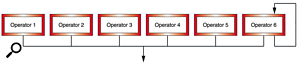Figure 11: 6-operator FM: algorithm 32 from a Yamaha DX7.In contrast, Figure 12 shows a more complex arrangement. If you're familiar with Yamaha's 1980s digital implementation of FM (as seen on their DX synths, to name just one series), you should recognise such diagrams, as each digital Yamaha FM keyboard synth had all its possible algorithms printed on its top panel. Reading these algorithm diagrams is easy, as long as you remember that the operators acting as Carriers are always on the bottom row of the diagrams, with the Modulators arranged above them.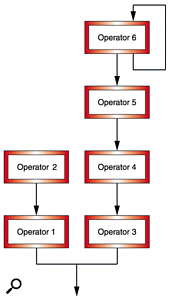Figure 12: 6-operator FM: algorithm 1 from a Yamaha DX7.In Figure 12, therefore, Operator 2 is acting as Modulator for Operator 1 (a Carrier) while, at the same time, Operator 6 is modulating Operator 5 which is modulating Operator 4, which is modulating Operator 3, which is another Carrier. The outputs from the two Carriers are then summed together using a simpler mixer, and you hear the combined results of the two signal-generation paths. The benefits of this arrangement are, I hope, obvious. For example, you can create an evolving sustained tone using Operators 1 and 2, and use Operators 3, 4, 5 and 6 to add a complex 'attack' partial at the start, thus giving the sound life and character.

Figure 12 raises two important further points. The first is that you can cascade Operators, so there is nothing stopping you from modulating Modulators. The second is that you can mix the outputs from Carriers, as they are just conventional audio signals — so you can treat them as partials within the sound.

There are two other configurations you can use as building blocks in your FM patches, and I have shown these in Figures 13 and 14. The first is that of two Modulators modulating a single Carrier. You might think that this would lead to unpredictable results, but in practice you obtain the arithmetic sum of the two predicted waveforms. To make that statement clearer: if Operator 2 (a Modulator) and Operator 1 (the Carrier) would in isolation produce a square wave, and Operator 3 (another Modulator) and the Carrier would produce a sawtooth wave, the output from Figure 13 would look like a square wave and a sawtooth mixed together.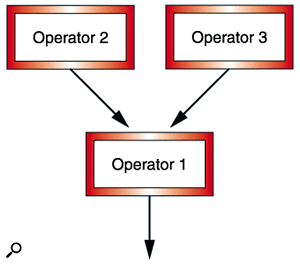Figure 13: Multiple Operators affecting a single Carrier.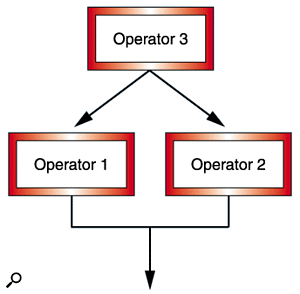Figure 14: A single Operator affecting multiple Carriers.The second configuration is that of one Modulator simultaneously affecting two Carriers (see Figure 14). In this case Operator 3 (the Modulator) acts independently on Operators 1 and 2 (the Carriers) in just the way it would if it were modulating only one of them. The results of these are added together by a simple audio mixer.

## Feedback

You'll have noticed that some of the operators in these diagrams show outputs that loop back on themselves. These, for obvious reasons, are called feedback loops, and they dramatically change the nature of the operator. Let's consider the operator in Figure 15, and say that this is producing a sine wave of 100Hz. Then, following our reasoning above, it is also receiving a 100Hz sine wave (its own output) as a Modulator, thus making it produce a complete harmonic series at its output. You can then use an input level control or a VCA within the feedback loop to control the brightness of the output waveform. Neat, huh?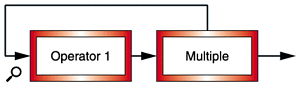Figure 15: Feedback in an FM system turns a sine wave generator into a sawtooth generator.Finally, let's prove that FM synthesis is not just the preserve of the DX-series Yamaha synths. Figure 16 shows a dual-operator feedback configuration that doesn't exist within Yamaha's FM system, but is simple to patch on a modular analogue synth.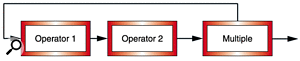Figure 16: Feedback in a 2-operator FM system turns two sine wave generators into a noise source.As you can see, there are two operators, and the output of the second, modulated operator is fed back to the input of the first operator. If the frequencies of the two operators are identical, this is no different from the configuration shown in Figure 15. But what happens if their frequencies are different and not related by integers? Now you have trouble! Look at it this way... the sine wave output by Operator 1 produces FM side bands in the output of Operator 2. This complex spectrum is then fed back to Operator 1, producing a hugely complex spectrum that is itself then fed to Operator 2, further complicating the output spectrum. This complex spectrum is then fed back to Operator 1, producing a hugely complex spectrum that is itself then fed to Operator 2, further complicating the output spectrum... As you might imagine, the output soon includes thousands — even millions — of frequencies, and deteriorates almost instantly into noise. This makes analogue FM an unlikely, but hugely powerful generator of drum and percussion sounds (refer back to Part 2 of this series if I've lost you).

## Conclusion

For some people, the last two months of this series may have looked like a DX7 tutorial, and it's true that everything I have written (except for the last example above) is applicable to this instrument, plus the dozens of other digital FM synthesizers produced by Yamaha, Korg and Elka. But that's to miss the point... everything I have written is applicable to any synthesizer that lets you direct the output of one oscillator to the pitch CV input of another. That includes the ARP 2600, the Minimoog (switch VCO3 to modulate VCO1 at audio frequencies), and the EMS VCS3, not to mention many other monosynths, DCO-based polysynths with 'cross modulation' or FM capabilities and, of course, all freely patchable modular synths.

So don't ignore FM — it's a powerful part of analogue synthesis. What's more, it produces sounds that conventional forms of subtractive synthesis cannot, filling holes in your sonic armoury that you never knew you had.